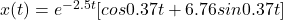## A mass with mass 4 is attached to a spring with spring constant 25.5625 and a dashpot giving a damping 20. The mass is set in motion with in

Question

A mass with mass 4 is attached to a spring with spring constant 25.5625 and a dashpot giving a damping 20. The mass is set in motion with initial position 1 and initial velocity 0. (All values are given in consistent units.) Find the position function x(t):

in progress 0
2 months 2021-07-30T03:05:02+00:00 1 Answers 4 views 0

1. m = 4

k = 25.56

b = 20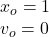The differential equation for damping motion is: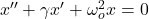where,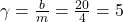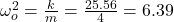Substitute the values in the differential equation and consider x” = r², x’ =r and solve: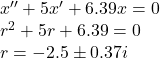Therefore, solution is given by: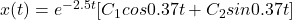$$\\$$

at t = 0, x = 1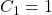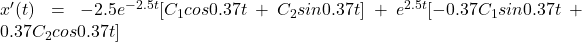at t = 0

x’ =0$$C_2=6.76$$